# ISEE Lower Level Quantitative : How to compare fractions

## Example Questions

1 2 3 4 6 Next →

### Example Question #51 : How To Compare Fractions

Select the symbol to correctly fill in the blank below.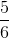__________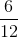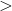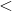Explanation:

To compare fractions, we need to first make common denominators.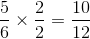Now that we have common denominators, we can compare numerators. The fraction with the bigger numerator has the greater value.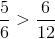### Example Question #52 : How To Compare Fractions

Select the symbol to correctly fill in the blank below.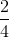__________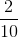Explanation:

To compare fractions, we need to first make common denominators.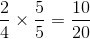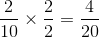Now that we have common denominators, we can compare numerators. The fraction with the bigger numerator has the greater value.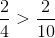### Example Question #53 : How To Compare Fractions

Select the symbol to correctly fill in the blank below.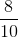__________Explanation:

To compare fractions, we need to first make common denominators.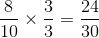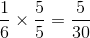Now that we have common denominators, we can compare numerators. The fraction with the bigger numerator has the greater value.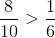### Example Question #54 : How To Compare Fractions

Select the symbol to correctly fill in the blank below.__________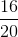Explanation:

To compare fractions, we need to first make common denominators.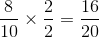Now that we have common denominators, we can compare numerators. The fraction with the bigger numerator has the greater value.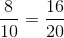### Example Question #55 : How To Compare Fractions

Select the symbol to correctly fill in the blank below.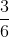__________Explanation:

To compare fractions, we need to first make common denominators.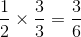Now that we have common denominators, we can compare numerators. The fraction with the bigger numerator has the greater value.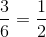### Example Question #56 : How To Compare Fractions

Select the symbol to correctly fill in the blank below.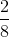__________Explanation:

To compare fractions, we need to first make common denominators.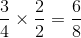Now that we have common denominators, we can compare numerators. The fraction with the bigger numerator has the greater value.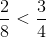### Example Question #57 : How To Compare Fractions

Select the symbol to correctly fill in the blank below.__________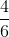Explanation:

To compare fractions, we need to first make common denominators.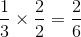Now that we have common denominators, we can compare numerators. The fraction with the bigger numerator has the greater value.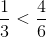### Example Question #58 : How To Compare Fractions

Select the symbol to correctly fill in the blank below.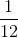__________Explanation:

To compare fractions, we need to first make common denominators.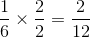Now that we have common denominators, we can compare numerators. The fraction with the bigger numerator has the greater value.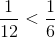1 2 3 4 6 Next →

### All ISEE Lower Level Quantitative Resources